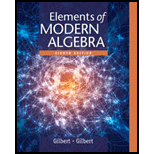# Use Exercise 25 and generalized induction to prove that ( n r ) is an integer for all n and r with 0 ≤ r ≤ n 25 . Let a and b be a real number, and let n be integers with 0 ≤ r ≤ n . The binomial theorem states that ( a + b ) n = ( n 0 ) a n + ( n 1 ) a n − 1 b + ( n 2 ) a n − 2 b 2 + ... + ( n r ) a n − r b r + ....... + ( n n − 2 ) a 2 b n − 2 + ( n n − 1 ) a b n − 1 + ( n n ) b n = ∑ r = 0 n ( n r ) a n − r b r Where the binomial coefficients ( n r ) are defined by ( n r ) = n ! ( n − r ) ! r ! , With r ! = r ( r − 1 ) ......... ( 2 ) ( 1 ) for r ≥ 1 and 0 ! = 1 . Prove that the binomial coefficients satisfy the equation ( n r − 1 ) + ( n r ) = ( n + 1 r ) for 1 ≤ r ≤ n This equation generates all the “"interior “"entries (printed in bold) of Pascal’'s triangle. 1 1 1 1 2 1 1 3 3 1 1 4 6 4 1 1 5 1 0 1 0 5 1 ⋰ ⋮ ⋱### Elements Of Modern Algebra

8th Edition
Gilbert + 2 others
Publisher: Cengage Learning,
ISBN: 9781285463230### Elements Of Modern Algebra

8th Edition
Gilbert + 2 others
Publisher: Cengage Learning,
ISBN: 9781285463230

#### Solutions

Chapter
Section
Chapter 2.2, Problem 26E
Textbook Problem

## Use Exercise 25 and generalized induction to prove that (       n       r ) is an integer for all n and r with 0 ≤ r ≤ n 25     . Let a and b be a real number, and let n be integers with 0 ≤ r ≤ n . The binomial theorem states that ( a + b ) n = ( n 0 ) a n + ( n 1 ) a n − 1 b + ( n 2 ) a n − 2 b 2 + ... + ( n r ) a n − r b r + ....... + (       n n − 2 ) a 2 b n − 2 + ( n n − 1 ) a b n − 1 + ( n n ) b n = ∑ r = 0 n ( n r )     a n − r b r Where the binomial coefficients ( n r ) are defined by ( n r ) = n ! ( n − r ) ! r ! ,With r ! = r ( r − 1 ) ......... ( 2 ) ( 1 ) for r ≥ 1 and 0 ! = 1 . Prove that the binomial coefficients satisfy the equation (     n r − 1 ) + ( n r ) = ( n + 1       r ) for 1 ≤ r ≤ n This equation generates all the “"interior “"entries (printed in bold) of Pascal’'s triangle. 1 1 1 1 2 1 1 3 3 1 1 4 6 4 1 1 5 1 0 1 0 5 1 ⋰ ⋮ ⋱

Expert Solution
To determine

To prove: (nr) is an integer for all integers n and r with 0rn by using generalized induction.

### Explanation of Solution

Given information:

Let a and b are real numbers, and let n and r be integers with 0rn, the binomial theorem states that,

(a+b)n=(n0)an+(n1)an1b+(n2)an2b2++(nr)anrbr++(nn)bn

=r=0n(nr)anrbr

Where the binomial coefficients (nr) are defined by (nr)=n!(nr)!r!

with r!=r(r1)(2)(1) for r1 and 0!=1.

Formula used:

(i) Strategy: Proof by Generalized Induction

1. Basic Step: The statement is verified for n=a.

2. Induction Hypothesis: The statement is assumed true for n=k, where ka.

3. Inductive Step: With this assumption made, the statement is then proved to be true for n=k+1.

(ii) (nr1)+(nr)=(n+1r) for 1rn.

Proof:

Consider the statement, “ (nr) is an integer for all integers n and r with 0rn

For r=0

By using (nr)=n!(nr)!r!,

(n0)=n!(n0)!0!

As 0!=1,

(n0)=n!(n)!1=1

Thus, (n0)=1

Therefore, the statement is true for r=0

### Want to see the full answer?

Check out a sample textbook solution.See solution

### Want to see this answer and more?

Bartleby provides explanations to thousands of textbook problems written by our experts, many with advanced degrees!

See solution